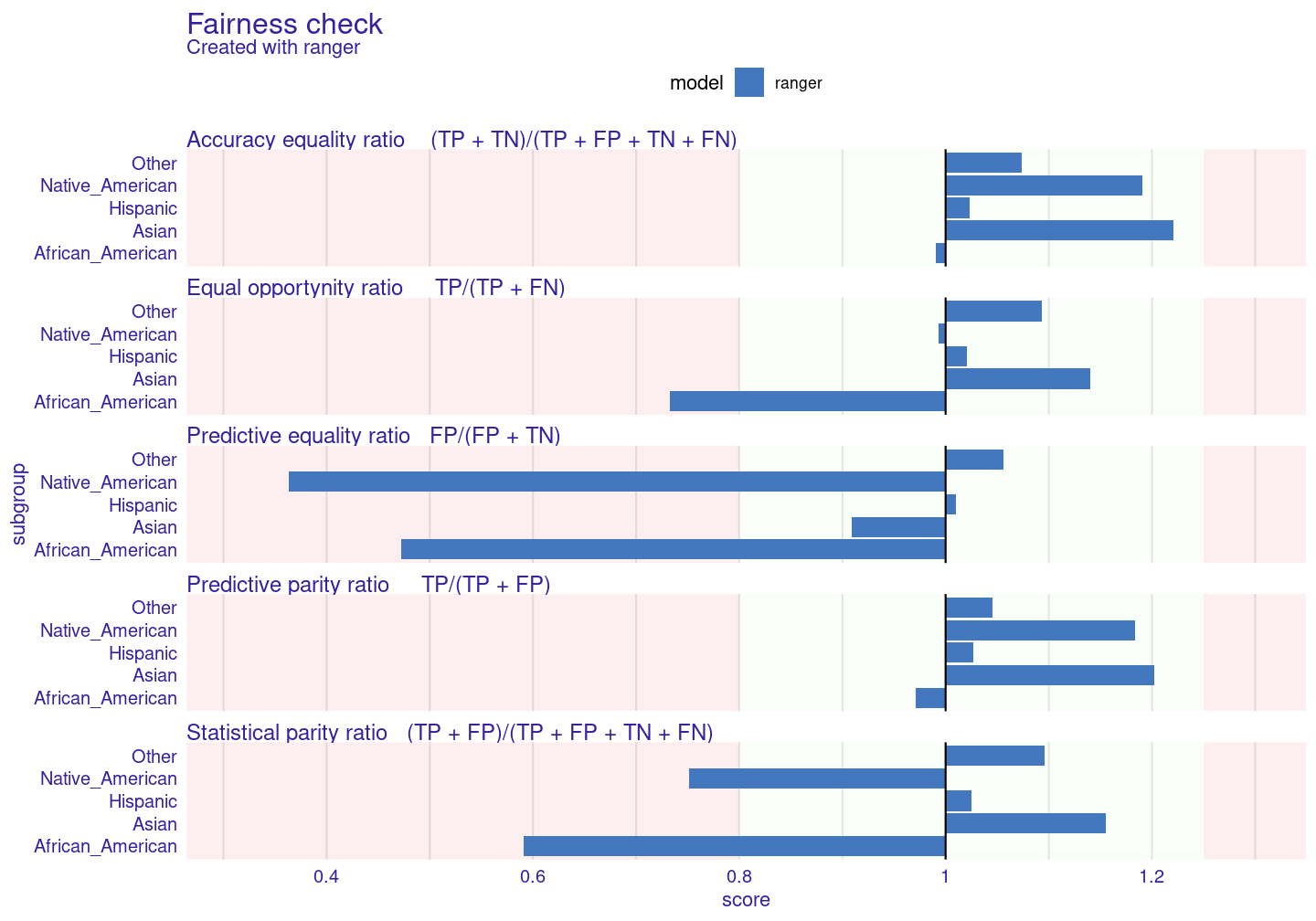# 1 fairmodels

In this tutorial you will get to know when, why and how to use `fairmodels`. `fairmodels` is a tool for bias detection, visualization and mitigation. It is compatible with `DALEX` and `DALEXtra` which are model agnostic explainers. Some knowledge of how to use those explainers will be needed but in this tutorial you should grasp the idea.

For this tutorial we will use `compas` data to see if someone will become recidivist in next 2 years.

## 1.1 Why?

Let’s say you are building algorithm for court system that predicts if someone will become recidivist in the future. First you gather information then you build a model and predict outcomes. You get satisfying accuracy. It is pretty good, but it appears that the model is more likely to say that African Americans will become recidivists. It was trained on data that where discrimination of certain ethnic groups was visible. Racist algorithms are no good for the court system. So now we have some options. First one is to gather more reliable data, and the second one is trying to understand bias and choose model with least amount of it. We will choose the second option.

## 1.2 Data

We will use modified ProPublica’s compas data to represent our problem.

``````library(fairmodels)
data("compas")

For `fairmodels` package to work properly we want to flip factor levels in target variable, so positive outcome (not being a recidivist) is being predicted by models. It is only needed for one specific function but more on it later.

``compas\$Two_yr_Recidivism <- as.factor(ifelse(compas\$Two_yr_Recidivism == '1', '0', '1'))``

## 1.3 Basic features

We train a `ranger` model and create an explainer with `DALEX`.

``````library(DALEX)
library(ranger)

# train
rf_compas <- ranger(Two_yr_Recidivism ~., data = compas, probability = TRUE)

# numeric target values
y_numeric <- as.numeric(compas\$Two_yr_Recidivism)-1

# explainer
rf_explainer <- explain(rf_compas, data = compas[,-1], y = y_numeric, colorize = FALSE)``````
``````#> Preparation of a new explainer is initiated
#>   -> model label       :  ranger  (  default  )
#>   -> data              :  6172  rows  6  cols
#>   -> target variable   :  6172  values
#>   -> predict function  :  yhat.ranger  will be used (  default  )
#>   -> predicted values  :  numerical, min =  0.1590342 , mean =  0.5449543 , max =  0.8705104
#>   -> model_info        :  package ranger , ver. 0.12.1 , task classification (  default  )
#>   -> residual function :  difference between y and yhat (  default  )
#>   -> residuals         :  numerical, min =  -0.8505374 , mean =  -7.418443e-05 , max =  0.7780252
#>   A new explainer has been created!``````

### 1.3.1 fairness check

Than we create call function `fairness_check()` This function aggregates many explainers so you may compare many models. We assign object to name `fobject` which is short version of `fairness_object` - object returned by `fairness_check()`,

``````fobject <- fairness_check(rf_explainer,                         # explainer
protected = compas\$Ethnicity,         # protected variable as factor
privileged = "Caucasian",             # level in protected variable, potentially more privileged
cutoff = 0.5,                         # cutoff - optional, default = 0.5
colorize = FALSE)                         ``````
``````#> Creating fairness object
#> -> Privileged subgroup       : character ( Ok  )
#> -> Protected variable        : factor ( Ok  )
#> -> Cutoff values for explainers  : 0.5 ( for all subgroups )
#> -> Fairness objects      : 0 objects
#> -> Checking explainers       : 1 in total (  compatible  )
#> -> Metric calculation        : 12/12 metrics calculated for all models
#>  Fairness object created succesfully``````

Let’s see if our ranger model has bias.

``print(fobject, colorize = FALSE)``
``````#>
#> Fairness check for models: ranger
#>
#> ranger passes 2/5 metrics
#> Total loss:  3.7895``````
``plot(fobject)``Of course the `protected` parameter can be changed, for example to (here binary) `compas\$Sex`.

In many metrics `ranger` exceeds fairness threshold (which can be changed by `epsilon` parameter). If bars reach red field on the left it means that there is bias towards certain unprivileged subgroup. If they reach one on the right it means bias towards privileged (Caucasian - in all metrics here Caucasian subgroup is referenced as base - 1) subgroup. This values mean the proportion (ratio) of certain unprivileged subgroup to privileged subgroup (eg. Asian/Caucasian). By default it represents 80% rule (for eg. women should get credit at rate at least 80% of that of men) . Metrics measure how equal treatment and mistreatment among subgroups is. More on those metrics: wikipedia

Why do we have this bias? Model did learn from biased data. We can see it on plot below

### 1.3.2 plot density

``plot_density(fobject)``